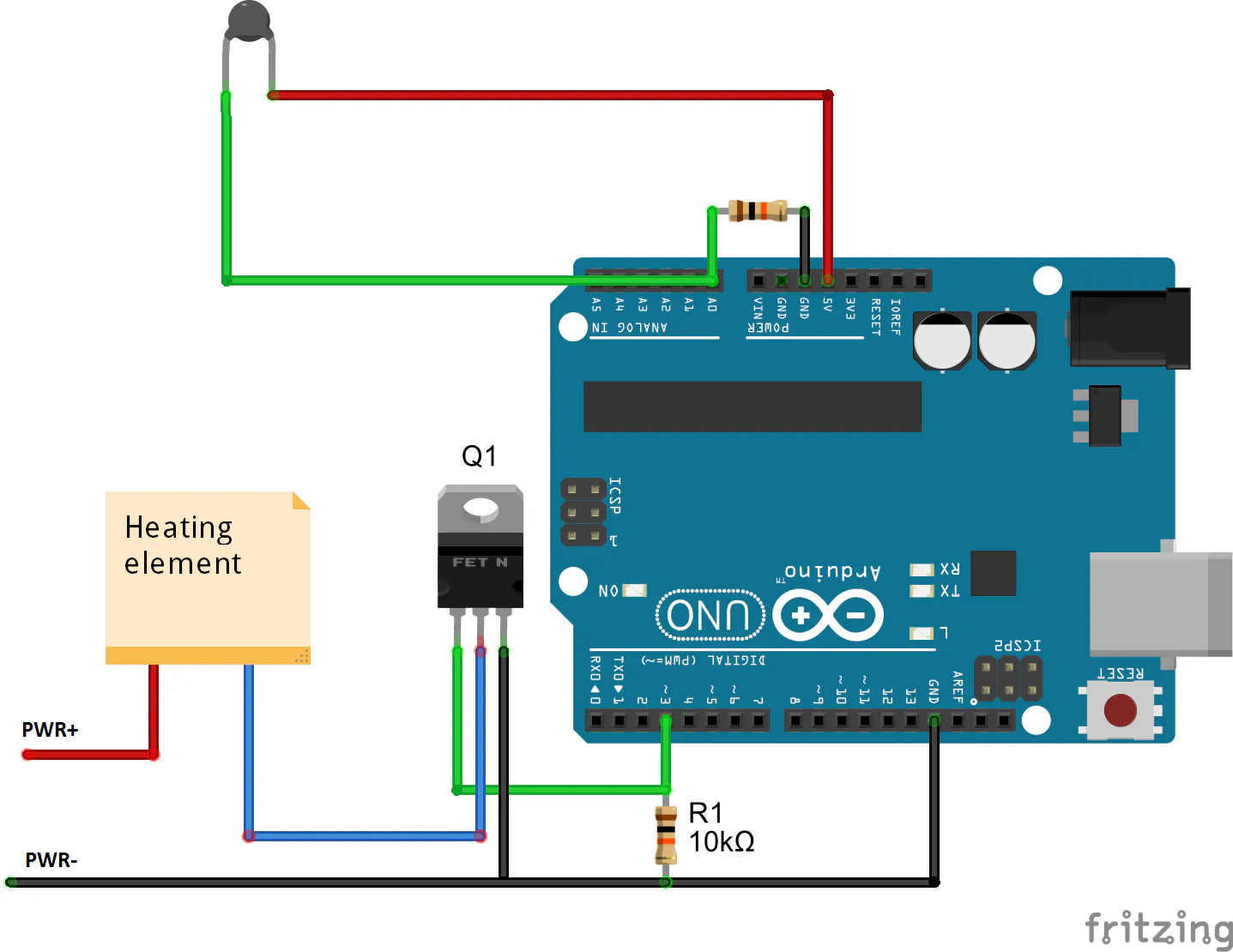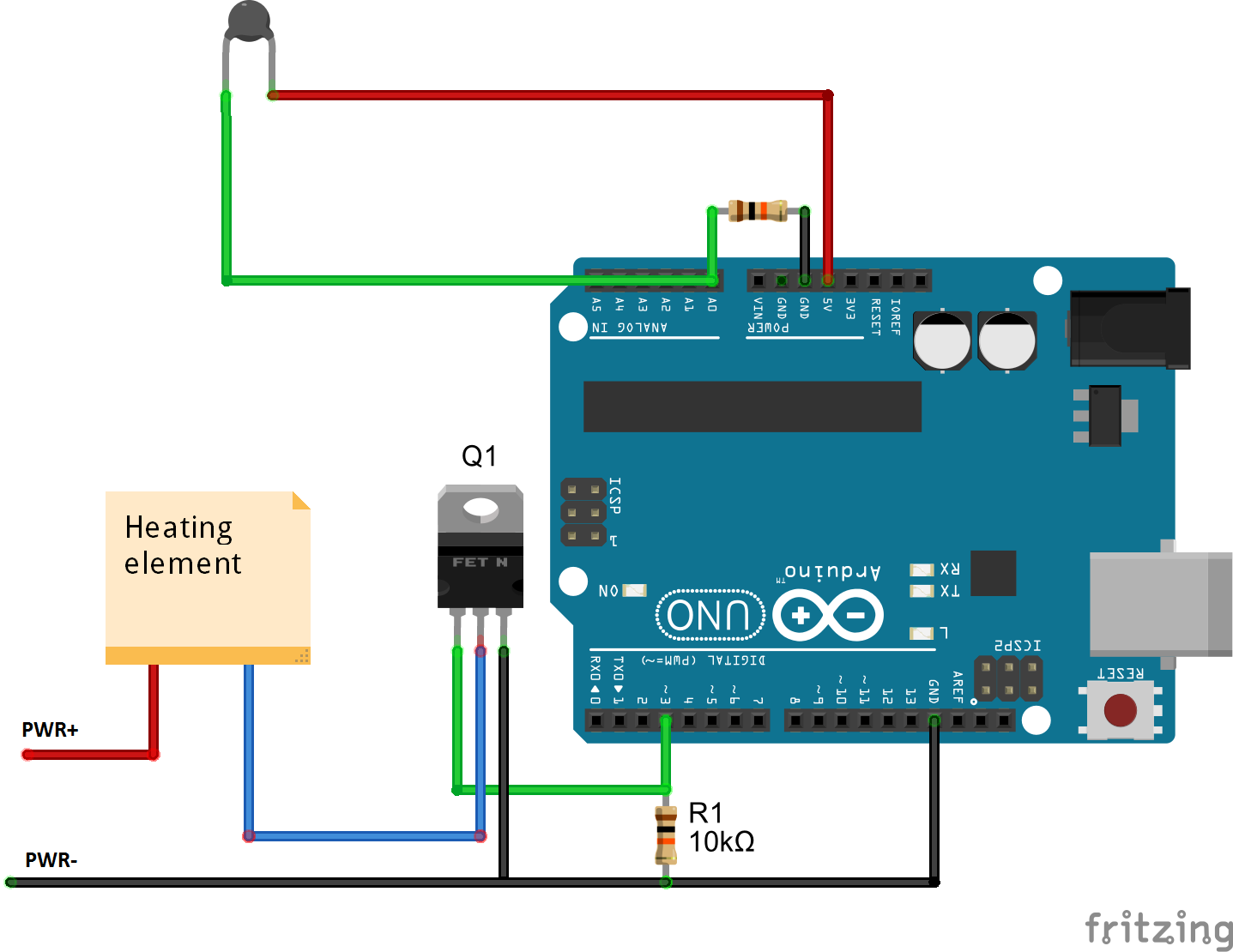Project showcaseMini-thermostat with input for a 2-wire NTC thermistor and output for a resistive heating element. Plus code & schematics.

• 10,445 views
• 8 respects

## Necessary tools and machinesSoldering iron (generic)

This device is a simplified version of the prototype used in an R&D collaboration between Tallinn University of Technology and Selfdiagnostics Deutschland GmbH. For more information, please refer to this paper: https://www.witpress.com/elibrary/cmem/5/1/1602.

For You, this project can serve as a general purpose mini-thermostat. You can easily use it with 2-wire 10k NTC thermistors and resistive heaters.

### What you'll need:

• soldering equipment
• basic understanding of electronics & programming

## Code

##### Temperature controllerC/C++
Complete temperature controller code. Just copy and paste.

Includes:
- thermistor code for temperature readout
- code for controlling heater input
- basic PID controller
- basic serial interface
```// variables and definitions

//Important parameter, set to match environment
const int dt = 500; // [ms] time constant in milliseconds (controller clock rate = 1/(dt/1000) [Hz])
#define SetTemp 62.8 // [degC] set temperature in DegC
#define MinTemp 20 // [degC] minimum expected temperature (needed for rescaling inputs)
#define MaxTemp 65 // [degC] maximum allowed temperature, over which heater is turned off (needed for rescaling inputs)
int SetTime = 1800; // [s] timer in seconds, if reached, running stops [Default: 1800]

//I/O pins - don't edit unless replaced
#define thermistorPin A0
#define FETPin 3
//#define LEDPin //number of LED pin (optional)

//control parameters - editing not recommended
double K_P_ctrl = 15; //proportional gain
double K_I_ctrl = 0; //integral gain (set to lower values i.e. 10^-3)
double K_D_ctrl = 0; //derivative gain

#include <math.h>

//Inititalization
//target temperature reached?
bool bInRange = 0;

//ticks per ms
int TicksPerMS = floor(1000/dt);

//Initialize PID variables:
float previous_error = 0;
float s_integral = 0;

//Thermistor code
double Temp;
Temp = 1 / (0.001129148 + (0.000234125 + (0.0000000876741 * Temp * Temp ))* Temp );
Temp = Temp - 273.15;            // Convert Kelvin to Celcius
return Temp;
}

//PID controller code
void Control_PID(double iTemp){

//Overheat protection
if(iTemp>MaxTemp){
analogWrite(FETPin, 0);
Serial.println("Error:overheat. Heater turned off");
return;
}

//In range? If in range, maybe turn on LED?
if((iTemp) >= SetTemp){
if(bInRange==0){
//digitalWrite(LEDPin, HIGH);
bInRange=1;
}
}else{
if(bInRange==1){
//digitalWrite(LEDPin, LOW);
bInRange=0;
}
}

//PID subroutine
float err = SetTemp - iTemp;
//Serial.println(err);
s_integral += err*dt;
//Serial.println(s_integral);
float s_derivative = (err - previous_error)/dt;
//Serial.println(s_derivative);
int U_in_ctrl = (K_P_ctrl*err + K_I_ctrl*s_integral + K_D_ctrl*s_derivative)/(MaxTemp-MinTemp)*255;
previous_error = err;

// put voltage to output and write value to serial monitor
Serial.print("Output PWM frequency: ");

if (U_in_ctrl<=255){
if (U_in_ctrl > 0){
analogWrite(FETPin, U_in_ctrl);
Serial.println(U_in_ctrl);
}
else
{
analogWrite(FETPin, 1);
Serial.println("1 - cca. 0 V");
}
}
else{
analogWrite(FETPin,255);
Serial.println("255 - cca. 5 V");
}
}

void setup() {
Serial.begin(9600);
pinMode(FETPin, OUTPUT);
//pinMode(LEDPin, OUTPUT);

//rescale timer according to dt
SetTime = SetTime * TicksPerMS;
}

void loop() {
//Take a temperature reading and display it
Serial.print("Temperature:");
Serial.println(Temp);  // display temperature

//Timer serial out - displays time on serial monitor
Serial.print(SetTime/60*dt/1000);
Serial.print(" [mins] - SetTime: ");
Serial.print(SetTime);
Serial.println("");

//Call controller algorithm
Control_PID(Temp); // call controller algorithm

//End line in serial monitor...
Serial.println("");
Serial.println("");

//Timer ticking (countdown)
if (SetTime>0){
SetTime--;
// if zero reached
if (SetTime==0){

while(1) {
//loop until disconnected
Serial.println("Time ran out, controller stopped. Please disconnect or reset the controller.");
digitalWrite(FETPin, LOW);
delay(dt);
}
}
}

//wait dt before next cycle
delay(dt);
}
```
##### Heater control library CPPC/C++
Arduino library for heater control. You can include this in your own project.
```/****************************************************************
* Heater control library
*
*  Return codes:
*   1: in range
*   0: not in range
*   -1: error
*****************************************************************/

#include "Arduino.h"
#include "HeaterControl.h"

HeaterControl::HeaterControl(int FETPin)
{
pinMode(FETPin, OUTPUT);
_FETPin = FETPin;
}

int HeaterControl::Control_PID(double dCurrentTemperature, int dt, int ThresholdTemp, double MaxTemp, double MinTemp, double SetTemp, double K_P_ctrl, double K_I_ctrl, double K_D_ctrl)
{
bool bInRange =0;

//Overheat protection
if (dCurrentTemperature > MaxTemp) {
analogWrite(_FETPin, 0); //turn off heater
return -1; //return with overheat code
}

//In range? If yes, return with in range code
if (dCurrentTemperature >= ThresholdTemp && dCurrentTemperature < (ThresholdTemp + 2)) { bInRange = 1; }

//PID subroutine
float err = SetTemp - dCurrentTemperature;
s_integral += err * dt;
float s_derivative = (err - previous_error) / dt;
int U_in_ctrl = (K_P_ctrl * err + K_I_ctrl * s_integral + K_D_ctrl * s_derivative) / (MaxTemp - MinTemp) * 255;
previous_error = err;

// put voltage to output and write value to serial monitor
if (U_in_ctrl <= 255)
{
if (U_in_ctrl > 0) {
analogWrite(_FETPin, U_in_ctrl);
}
else
{
analogWrite(_FETPin, 1);
}
}
else
{
analogWrite(_FETPin, 255);
}

return bInRange;
}

void HeaterControl::TurnOff()
{
analogWrite(_FETPin, 0); //turn off heater
}
```
```/****************************************************************
*
*****************************************************************/

#ifndef HeaterControl_h
#define HeaterControl_h

#include "Arduino.h"

class HeaterControl
{
public:
HeaterControl(int FETPin);
int Control_PID(double dCurrentTemperature, int dt, int ThresholdTemp, double MaxTemp, double MinTemp, double SetTemp, double K_P_ctrl, double K_I_ctrl, double K_D_ctrl); //for ATMega328P
void TurnOff();

private:
int _FETPin;

//internal
float previous_error = 0; //Initialize PID variables
float s_integral = 0; //Initialize PID variables
};

#endif
```
##### Thermometer library CPPC/C++
Arduino library for thermistor input. You can include this in your own project
```#include "Arduino.h"
#include "Thermistor.h"

Thermistor::Thermistor(int ThermistorPin)
{
pinMode(ThermistorPin, INPUT);
thermistorPin = ThermistorPin;
}

double Thermistor::Thermistor_Read(int TemperatureOffset, double T0, double R0, double B)
{
//Thermistor code with a generalized equation
//Steinhart-Hart equation: T[K]=1/(a+b*log(R)+c*(log(R))^3)
//where a=1/T_0-(1/B)*log(R_0), b=1/B and c = 0

double Temp;

double R;
R = 10000.0 * (1024.0 / RawADC - 1);
Temp = 1 / (1 / T0 + 1 / B * log(R / R0));
Temp = Temp - 273.15;            // Convert Kelvin to Celsius
return Temp;
}
```
```/* Thermistor control library header
*/

#ifndef Thermistor_h
#define Thermistor_h

#include "Arduino.h"

class Thermistor
{
public:
Thermistor(int ThermistorPin); //constructor
double Thermistor_Read(int TemperatureOffset, double T0, double R0, double B);

private:
int thermistorPin;
};

#endif
```

## SchematicsMarch 9, 2017

#### Members who respect this project

See similar projects
you might like

#### Smart Thermostat

Project tutorial by Arduino_Genuino

• 91,719 views
• 105 respects

#### Servo Control with TV Remote Control

Project showcase by eldo85

• 23,440 views
• 46 respects

#### Programmable Pocket Power Supply with OLED Display

Project tutorial by Shahariar

• 13,576 views
• 47 respects

Project tutorial by Giovanni Carrera

• 11,276 views
• 10 respects

#### Hacking Qualcomm (Quick Charge) QC 2.0/3.0 With ATtiny85

Project tutorial by Shahariar

• 30,864 views